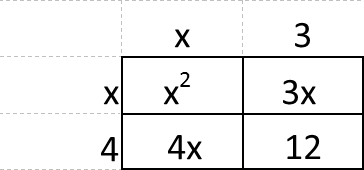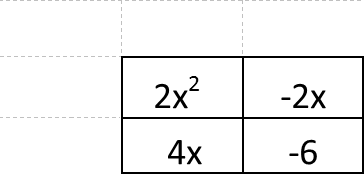top of page

# New Math Series Part 1: FactoringHello all! In this series I am going to be discussing a few of the ways that math has changed since I was in school. For some context, I am 30. I graduated from high school in 2004, and from the University of Oregon with a BA in Mathematics and a Secondary Education emphasis in 2008. A lot of changes have happened in Oregon's math curriculum since then, with the adoption of common core educational standards and mandatory testing placed as a graduation requirement. Some of the changes in curriculum have been good, some have been bad, but all of them are changes none the less! The purpose of this series is to familiarize parents with a few of the "big picture" changes I have seen in my 11 years as a private tutor so they can broaden their understanding of their students' homework and classwork.

One of the biggest changes I have seen in the time since I graduated has been in factoring. I also feel like this is a good topic to use to start off the series because it has been a positive change! We didn't have product squares, and the diamond method was nothing I had ever heard of when I learned how to factor (cue the "back in my day" old-timey rant).

Here's how factoring would look when I was in school:As you can see, we would guess at the factors of the first and last term and use those to create factored terms. Then we had to test each factored term pair (using FOIL) to determine which was yielded the correct middle term. It. Was. Terrible. It's no wonder so many people didn't like factoring! Unfortunately this is an incredibly important concept, and one that is critical for students who want to move forward into higher math. How many young people might have been discouraged from pursuing careers that required an excursion into mathematics simply because of concepts like this that were slow and cumbersome for very little yield?

Now let's discuss the new way of factoring.

To understand this, you will need to know a little bit about a product square.

A product square is a visual representation of the process we call FOIL. FOIL stands for First, Inside, Outside, Last, and is in place to remind us how to multiply together two sums. When multiplying (x+4) and (x-7), we have to make sure every pair of items gets multiplied: first (x and x), outside (x and -7), inside (4 and x), last (4 and -7).

A product square does the same thing, just a lot more simply. To illustrate this, we will first look at how a product square is used to multiply large numbers, like 34 x 12. To simplify the process we think of the problem as (30+4)(10+2). Now we construct squares (really rectangles, I know) with side lengths that correspond to our values. We don't have to make them proportional, as long as we can conceptualize the idea of rectangles with these dimensions.Now we can perform our multiplication. Think of multiplication as finding the area of a box. When we perform the operation "5 times 2" we are really just finding the area of a rectangle that is 5 units long and 2 units wide. In the above box, we are finding the areas of four rectangles, each of them with the dimensions listed along their edges.The final answer, then is the sum 300+60+40+8, or 408. That's how we find 34x12 without a calculator!

For algebraic expressions the process is similar. To multiply (x-2)(3x+5) we would set it up like so:Now the final product is just : 3x^2 + 5x - 6x -10 = 3x^2 -x -10 after we combine like terms.

The second way to use a product square is by moving backwards, from the "FOIL-ed" inside to the terms on the outside.Here we have to work back to the original values that would have yielded these terms. The easiest place to start is with the question, "What two terms, when multiplied together, make x-squared?" The answer is, of course, x and x. Once we have the first term on each side we can use the linear terms (4x and 3x) to determine the last terms on the outside.This tells us that the expression x^2 + 4x + 3x +12 = (x+3)(x+4). We also know that

x^2 + 4x + 3x +12 = x^2 + 7x +12, so we now know that the factored form of x^2 + 7x +12 is (x+3)(x+4).

The problem with this method arises when our middle term is not already split up for us. In the previous problem, we were given 3x and 4x instead of 7x. This told us how the middle should be split up so we didn't have to guess. If we hadn't known that the 7 needed to be split up this particular way we would have been left guessing. Our terms could have been x and 6x, 5x and 2x, 10x and -3x......the list goes on and on! What, then, do we do when presented with a polynomial like 2x^2 - x - 6? We know the leading term and the constant term need to go in as follows:However, there are infinite ways that the other two terms can be written. All we know about them is that they need to add to -x. They could be 4x and -5x, 11x and -12x, 0 and -x, or any other of infinite possibilities. How could we possibly know which pair to pick?!

In order to answer that questions, we need to move on to our second tool.

The second tool we need to have for factoring the "new" way is the infamous diamond. What do diamonds have to do with math, you ask? This will be fun!

This is what we refer to as a diamond:I am aware that it doesn't look like a diamond. The original shape was much more diamond-ish, but this is a much easier form to replicate, both on paper and by keyboard, so this is the form I have been using for a while now. The important thing is not the shape. The important thing is the placement of each number. The numbers on the left and right add together to make the bottom number and multiply to make the top number. This is always the case in a diamond.

Here is another example:Now let's see what happens when we are not given the whole diamond. Fill in the missing information in the diamond below:Did you get it? Your diamond should look like this:What happens, though, if you are not given both sides of the diamond?In this case we don't know what the right-hand value is. However, we do know that whatever it is, it must equal 12 when multiplies by 2. The only number that satisfies this condition is 6. If the right-hand value is 6, then the bottom value must be 8, so we get the solved diamond:The kind of diamond we will be using the most is the kind wherein the top and bottom are given and we are asked to find the side values.This diamond wants to know what two numbers multiply to make 6 and add to make 5. After a moment of thought, we say "2 and 3." It doesn't matter which value goes on which side; they are interchangeable.Now we will tie this concept back into our product squares from the beginning of the post.

When we were setting our quadratic polynomials up inside our product squares, we ran into the problem of not knowing how to break up the middle term. Diamonds are the solution to that problem.

In the top of the bottom we put the product of the leading and contact coefficients. Confusing? It doesn't have to be! This just means we multiply the coefficient of our squared term and our constant term (the one that doesn't have any x with it). This becomes the top of our diamond. The bottom of our diamond is the coefficient of the linear term, that is to say, the term that is multiplied by a variable with a power of 1. In many cases we refer to this as our "middle term."

Let's look at an example, such as 2x^2 + 4x - 6.

Our diamond is constructed as follows:Or:Now we are looking for two values that multiply to make -12 and add to make 4. These values are:Now we move into our product square. The diamond told us that our middle term should be split up as 6x and -2x. This gives us the product square:Now that we know all of the interior terms we can complete the outside of the square.